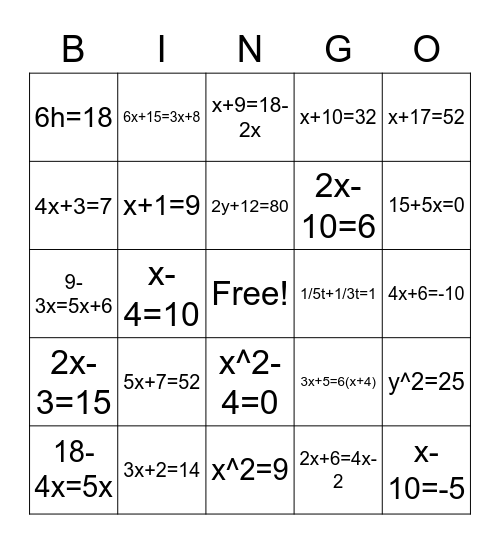# Math Bingo for Middle SchoolThis bingo card has a free space and 24 words: 5x+7=52, 3x+2=14, 2y+12=80, 18-4x=5x, x+17=52, 6h=18, 4x+3=7, 6x+15=3x+8, 1/5t+1/3t=1, x^2-4=0, 2x-10=6, 9-3x=5x+6, 3x+5=6(x+4), y^2=25, x+1=9, 2x+6=4x-2, 4x+6=-10, x+9=18-2x, 15+5x=0, x-4=10, x-10=-5, 2x-3=15, x+10=32 and x^2=9.

## Play Online